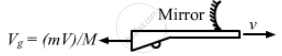Advertisement Remove all ads

# A Gun of Mass M Fires a Bullet of Mass M with a Horizontal Speed V. the Gun is Fitted with a Concave Mirror of Focal Length F Facing Towards the Receding - Physics

Sum

A gun of mass M fires a bullet of mass m with a horizontal speed V. The gun is fitted with a concave mirror of focal length f facing towards the receding bullet. Find the speed of separation of the bullet and the image just after the gun was fired.

Advertisement Remove all ads

#### Solution

Given,
The focal length of the concave mirror is f and M is the mass of the gun. Horizontal speed of the bullet is V.Let the recoil speed of the gun be Vg
Using the conservation of linear momentum we can write,
MV_g = mV
⇒ V_g =  m/M V
Considering the position of gun and bullet at time t = t,
For the mirror, object distance, u = − (Vt + Vgt)
Focal length, f = − f
Image distance, v = ?
Using Mirror formula, we have:
1/v + 1/u = 1/f

⇒ 1/v + 1/u = 1/f

⇒ 1/v = 1/-f - 1/u
⇒  1/v = -1/f + 1/ ( Vt + V_ g t)

⇒ 1/v = (-(Vt + V_g t) + f)/( (Vt + V_g t) f

⇒ v = (-(Vt - V _g t )  tf) / ( f - (V + Vg) t)
The separation between image of the bullet and bullet at time is given by:

v = u -(( V + V_g )tf )/ ( f- ( V + V g) t + ( V + Vg ) t

= (V + Vg ) t [ f/ (f-(V + Vg )t) + 1]

= 2( 1 + m/M )Vt
Differentiating the above equation with respect to 't' we get,
d ( v -u ) = 2 ( 1 + m/M ) V
Therefore, the speed of separation of the bullet and image just after the gun was fired is
2 ( 1 + m/M ) V.

Concept: Concave Mirror
Is there an error in this question or solution?
Advertisement Remove all ads

#### APPEARS IN

HC Verma Class 11, 12 Concepts of Physics 1
Chapter 18 Geometrical Optics
Q 76 | Page 416
Advertisement Remove all ads

#### Video TutorialsVIEW ALL 

Advertisement Remove all ads
Share
Notifications

View all notifications

Forgot password?